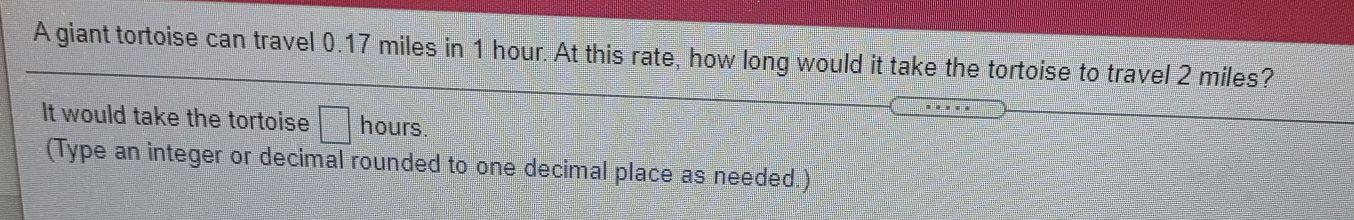### ¿Todavía tienes preguntas de matemáticas?

Pregunte a nuestros tutores expertos
Algebra
PreguntaA giant tortoise can travel $$0.17$$ miles in $$1$$ hour. At this rate, how long would it take the tortoise to travel $$2$$ miles? It would take the tortoise $$\square$$ hours. (Type an integer or decimal rounded to one decimal place as needed.)